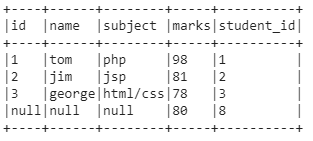# Concatenate Two & Multiple PySpark DataFrames (5 Examples)

This post explains how to concatenate two and multiple PySpark DataFrames in the Python programming language.

The article looks as follows:

• Introduction
• Creating Example Data
• Example 1: Concatenate two PySpark DataFrames using inner join
• Example 2: Concatenate two PySpark DataFrames using outer join
• Example 3: Concatenate two PySpark DataFrames using left join
• Example 4: Concatenate two PySpark DataFrames using right join
• Example 5: Concatenate Multiple PySpark DataFrames
• Video, Further Resources & Summary

With that, let’s get started:

## Introduction

PySpark is an open-source software that is used to store and process data by using the Python Programming language.

We can generate a PySpark object by using a Spark session and specify the app name by using the getorcreate() method.

`SparkSession.builder.appName(app_name).getOrCreate()`

After creating the data with a list of dictionaries, we have to pass the data to the createDataFrame() method. This will create our PySpark DataFrame.

`spark.createDataFrame(data)`

After that, we can present the DataFrame by using the show() method:

`dataframe.show()`

## Creating Example Data

In our case we are going to create three DataFrames: subjects, address, and marks with the student_id as common column among all the DataFrames.

1. Create subjects DataFrame

```# import the pyspark module import pyspark   # import the sparksession from pyspark.sql module from pyspark.sql import SparkSession   # creating sparksession and then give the app name spark = SparkSession.builder.appName('statistics_globe').getOrCreate()   #create a dictionary with 3 pairs with 3 values each #inside a list subjects = [{'id': '1', 'name': 'tom', 'subject': 'php'}, {'id': '2', 'name': 'jim', 'subject': 'jsp'}, {'id': '3', 'name': 'george', 'subject': 'html/css'}]       # creating a dataframe from the given list of dictionary subjects = spark.createDataFrame(subjects)   # display the final dataframe subjects.show()``````#create a dictionary with 3 pairs with 4 values each #inside a list address = [{'id': '1', 'name': 'tom', 'address': 'dallas'}, {'id': '2', 'name': 'jim', 'address': 'california'}, {'id': '3', 'name': 'george', 'address': 'dallas'}, {'id': '4', 'name': 'catherene', 'address': 'bihar'}]       # creating a dataframe from the given list of dictionary address = spark.createDataFrame(address)   address.show()```3. Create marks DataFrame

```#create a dictionary with 2 pairs with 4 values each #inside a list marks = [{'student_id': '1', 'marks': 98}, {'student_id': '2', 'marks': 81}, {'student_id': '3', 'marks': 78}, {'student_id': '8', 'marks': 80},]       # creating a dataframe from the given list of dictionary marks = spark.createDataFrame(marks)   marks.show()```Let’s see how to concatenate two and multiple DataFrames:

## Example 1: Concatenate two PySpark DataFrames using inner join

This example uses the join() function with inner keyword to concatenate DataFrames, so inner will join two PySpark DataFrames based on columns with matching rows in both DataFrames.

`dataframe1.join(dataframe2,dataframe1.column_name == dataframe2.column_name,"inner")`

In this example we are concatenating two PySpark DataFrames based on id column and the DataFrames are subjects and marks.

```#join two columns on marks and students dataframe based on student id column with inner join subjects.join(marks,subjects.id == marks.student_id,"inner").show(truncate=False)```## Example 2: Concatenate two PySpark DataFrames using outer join

This example uses the join() function with outer keyword to concatenate DataFrames, so outer will join two PySpark DataFrames based on columns with all rows (matching & unmatching) in both DataFrames.

`dataframe1.join(dataframe2,dataframe1.column_name == dataframe2.column_name,"outer")`

In this example we are concatenating two PySpark DataFrames based on id column and the DataFrames are subjects and marks.

```#join two columns on marks and students dataframe based on student id column with outer join subjects.join(marks,subjects.id == marks.student_id,"outer").show(truncate=False)```## Example 3: Concatenate two PySpark DataFrames using left join

This example uses the join() function with left keyword to concatenate DataFrames, so left will join two PySpark DataFrames based on the first DataFrame Column values matching with the Second DataFrame Column values.

`dataframe1.join(dataframe2,dataframe1.column_name == dataframe2.column_name,"left")`

In this example we are concatenating two PySpark DataFrames based on id column and the DataFrames are subjects and marks.

```#join two columns on marks and students dataframe based on student id column with left join subjects.join(marks,subjects.id == marks.student_id,"left").show(truncate=False)```## Example 4: Concatenate two PySpark DataFrames using right join

This example uses the join() function with right keyword to concatenate DataFrames, so right will join two PySpark DataFrames based on the second DataFrame Column values matching with the first DataFrame Column values.

`dataframe1.join(dataframe2,dataframe1.column_name == dataframe2.column_name,"right")`

In this example we are concatenating two PySpark DataFrames based on the id column and the DataFrames are subjects and marks.

```#join two columns on marks and students dataframe based on student id column with right join subjects.join(marks,subjects.id == marks.student_id,"right").show(truncate=False)```## Example 5: Concatenate Multiple PySpark DataFrames

This example uses the join() function to concatenate multiple PySpark DataFrames.

We can use the join() function again to join two or more dataframes.

For this, we have to specify the condition in the second join() function.

`dataframe1.join(dataframe2,["column_name"]) .join(dataframe3,dataframe1["column_name"] == dataframe3["column_name"])`

In this example we are concatenating the address PySpark DataFrame with the remaining two PySpark DataFrames based on the student id.

```#Join Multiple (3) daatrames based on student id column address.join(subjects,["id"]) .join(marks,address["id"] == marks["student_id"]) .show()```## Video, Further Resources & Summary

Do you need more explanations on how to join two and multiple PySpark DataFrames? Then you may have a look at the following YouTube video of the YouTube channel GeekCoders.